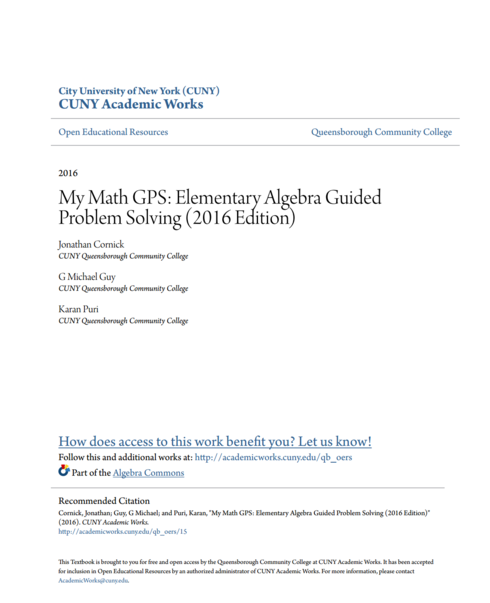# My Math GPS: Elementary Algebra Guided Problem Solving - 2016 Edition

(1 review)Jonathan Cornick, Queensborough Community College

Michael Guy, Queensborough Community College

Karan Puri

Language: English

## Conditions of UseAttribution-NonCommercial-ShareAlike
CC BY-NC-SA

## Reviews

Linear Equations And Inequalities

• GPS 1: Understanding Linear Equations
• GPS 2: Solving Linear Equations With Whole Numbers
• GPS 3: Adding Signed Numbers
• GPS 4: Subtracting Signed Numbers
• GPS 5: Multiplying And Dividing Signed Numbers
• GPS 6: Solving Linear Equations With Signed Numbers
• GPS 7: Translating Words Into Expressions And Equations
• GPS 8: Solving Linear Inequalities, Part 1
• GPS 9: Solving Linear Inequalities, Part 2
• GPS 10: Solving Linear Equations With Fractions
• GPS 11: More Linear Equations With Fractions
• GPS 12: Solving Literal Linear Equations

The Coordinate Plane And Lines

• GPS 13: Introduction To The Coordinate Plane
• GPS 14: Intercepts Of A Line
• GPS 15: Slope And Equations Of A Line
• GPS 16: The Slope-Intercept Equation Of A Line
• GPS 17: Slope-Intercept Equation And Graphing
• GPS 18: Graphing Lines In Slope-Intercept Form
• GPS 19: Finding An Equation Of A Line From Its Graph
• GPS 20: Horizontal And Vertical Lines

Systems Of Linear Equations

• GPS 21: Introduction To Systems Of Linear Equations And Solving Graphically
• GPS 22: More On Solving Systems Graphically
• GPS 23: Solving Systems Of Linear Equations Algebraically
• GPS 24: More On Solving Systems Algebraically

Exponents

• GPS 25: Rules Of Exponents, Part 1
• GPS 26: Rules Of Exponents, Part 2

Polynomials And Operations

• GPS 27: Introduction To Polynomials And Operations
• GPS 28: Multiplying Polynomials
• GPS 29: Removing The Greatest Common Factor
• GPS 30: Factoring By Grouping
• GPS 31: Factoring Trinomials By Grouping – Part 1
• GPS 32: Factoring Trinomials By Grouping – Part 2
• GPS 33: Factoring Trinomials By Grouping – Part 3
• GPS 34: Factoring Trinomials By Grouping – Part 4
• GPS 35: Factoring A Difference Of Squares
• GPS 36: Multistep Factoring
• GPS 37: Solving Quadratic Equations By Factoring

Algebraic Expressions

• GPS 38: Evaluating Algebraic Expressions

Square Roots And Operations

• GPS 39: Introduction To Square Roots
• GPS 40: Operations With Square Roots
• GPS 41: Pythagorean Theorem

## Ancillary Material

• Submit ancillary resource

My Math GPS: Elementary Algebra Guided Problem Solving is a textbook that aligns to the CUNY Elementary Algebra Learning Objectives that are tested on the CUNY Elementary Algebra Final Exam (CEAFE). This book contextualizes arithmetic skills into Elementary Algebra content using a problem-solving pedagogy. Classroom assessments and online homework are available from the authors.